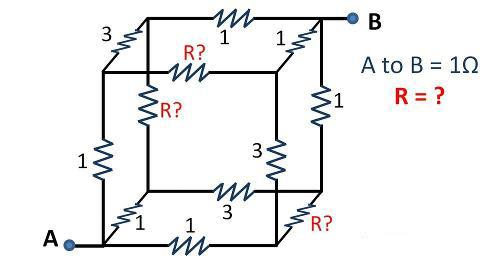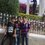R WoessssCan plzzz anyone give me step by step solution to this...Note by Aßhĩмanyu Singh
6 years, 4 months ago

This discussion board is a place to discuss our Daily Challenges and the math and science related to those challenges. Explanations are more than just a solution — they should explain the steps and thinking strategies that you used to obtain the solution. Comments should further the discussion of math and science.

When posting on Brilliant:

• Use the emojis to react to an explanation, whether you're congratulating a job well done , or just really confused .
• Ask specific questions about the challenge or the steps in somebody's explanation. Well-posed questions can add a lot to the discussion, but posting "I don't understand!" doesn't help anyone.
• Try to contribute something new to the discussion, whether it is an extension, generalization or other idea related to the challenge.
• Stay on topic — we're all here to learn more about math and science, not to hear about your favorite get-rich-quick scheme or current world events.

MarkdownAppears as
*italics* or _italics_ italics
**bold** or __bold__ bold

- bulleted
- list

• bulleted
• list

1. numbered
2. list

1. numbered
2. list
Note: you must add a full line of space before and after lists for them to show up correctly
paragraph 1

paragraph 2

paragraph 1

paragraph 2

> This is a quote
This is a quote
# I indented these lines
# 4 spaces, and now they show
# up as a code block.

print "hello world"
# I indented these lines
# 4 spaces, and now they show
# up as a code block.

print "hello world"
MathAppears as
Remember to wrap math in $$...$$ or $...$ to ensure proper formatting.
2 \times 3 $2 \times 3$
2^{34} $2^{34}$
a_{i-1} $a_{i-1}$
\frac{2}{3} $\frac{2}{3}$
\sqrt{2} $\sqrt{2}$
\sum_{i=1}^3 $\sum_{i=1}^3$
\sin \theta $\sin \theta$
\boxed{123} $\boxed{123}$

Sort by:

Using the methods outlined on this page: http://www.rfcafe.com/miscellany/factoids/kirts-cogitations-256.htm, you can set up the following equation:

$(\frac{1}{1 \ \Omega}+\frac{1}{1 \ \Omega}+\frac{1}{1 \ \Omega})^{-1} + (\frac{1}{3 \ \Omega}+\frac{1}{3 \ \Omega}+\frac{1}{3 \ \Omega}+\frac{1}{R}+\frac{1}{R}+\frac{1}{R})^{-1} +(\frac{1}{1 \ \Omega}+\frac{1}{1 \ \Omega}+\frac{1}{1 \ \Omega})^{-1} = 1 \ \Omega$.

Simplifying,

$(\frac{1}{3} \ \Omega) + (\frac{1}{1 \ \Omega} + \frac{3}{R})^{-1} + (\frac{1}{3} \ \Omega) = 1 \ \Omega$

$(\frac{1}{1 \ \Omega} + \frac{3}{R})^{-1} = \frac{1}{3} \ \Omega$

$\frac{1}{1 \ \Omega}+\frac{3}{R}=\frac{3}{1 \ \Omega}$

$\frac{3}{R}=\frac{2}{1 \ \Omega}$

$R=\frac{3}{2} \ \Omega$.

The following simulation shows that when a $5 \ \mathrm{V}$ voltage is applied across the circuit, a $5 \ \mathrm{A}$ current results, which implies the equivalent resistance of the circuit is $1 \ \Omega$, verifying that $R=\frac{3}{2} \ \Omega$ is correct: https://imgur.com/WoS0Yn7

- 6 years, 4 months ago

hey ricky is there any other method ????

- 6 years, 4 months ago

abe mujhe to ye method jyaada easy lag rha hai

- 6 years, 4 months ago

Look at the circuit, It is symmetric for any value of R. We can proceed to result in many ways including Kirchoff's law , One of the easiest method is given here . It can be only applied for a symmetric circuit(caution). Consider a 1 A current is flowing through it , as the circuit is symmetric 1/3 A will flow through each 1 ohm .Also 1/3 A will spit to 3 ohm and R, then finally 1/3 A will flow through last 1 ohm (1/3 A through each 1 ohm). Thus we get over all resistance as 1/3+(3 parallel to R)/3+1/3 =1 ,(3 parallel to R)/3=1/3 ,Thus R=3/2 . If any explanation needed, then please specify

- 6 years, 4 months ago

What will be the current in 3 ohm resistance?

- 6 years, 4 months ago

$\frac{1}{9} \ \mathrm{A} \approx 0.1111 \ \mathrm{A}$

- 6 years, 4 months ago

plzzz anyone

- 6 years, 4 months ago

sory where do you get that question

- 6 years, 4 months ago

u can use star & delta techniques that will simplify it

- 6 years, 4 months ago

There is a similar question in haliday,resnick and krane volume-2 physics book.Basically,here,you have to notice the symmetry and the points with the same potential.Through it,you can rearrange this circuit and solve it.Hopefully if i have not done any mistake in calculation the answer is 3/8.

- 6 years, 4 months ago

hey, actually i am in class 10th and we have not covered this topic yet.

- 6 years, 4 months ago

although i know abot v , i and parallel and series circuits

- 6 years, 4 months ago

Try applying Kirchoff's Law. By Conservation of Charge, the current entering at A should leave at B, right? So start there.

- 6 years, 4 months ago

plzzz can u give me step by step solution to this

- 6 years, 4 months ago

FIrst Reply.. u are in which standard and do you know Kirchoff Law

- 6 years, 4 months ago

i m in 10th standard, and according to cbse 10 physics syllabi, there is nothing as kirchoff law

- 6 years, 4 months ago

hey abhimanyu u can try to unferstand this. as points A and B are given name other 6 points as C,D,....H. Then try to figure out if a current say I starts from point A u can see the just next 3 corners are all connected by a 1 ohm resistor. so the current will be divided equally. then try to figure out those corners and the wires connecting then are they equal? try to find out the symmetry. U also can find from back ward also. after starting from A u try to picturise if the current I enters b and the next three corners of B are all equal resistance wire connected so the current would have come all equally from all those 3 corners try to ratioanalise ur thinking this way. i hope u will solve the remaining part urself.

- 6 years, 4 months ago

symertrycity will help u calculate the some point as same if distance from source and sink are same it will make easier

- 6 years, 4 months ago

1ohm

- 5 years, 7 months ago

From SOmewhere i got the hint that it's ans is 4/3

- 6 years, 4 months ago

is it 3/8?

- 6 years, 4 months ago

mantap, dapet soal darimananih?

- 6 years, 4 months ago

- 6 years, 4 months ago

okay, i'll

- 6 years, 4 months ago

that is indonesian language. iya mantap nih

- 6 years, 4 months ago

R=3 DUE TO SYMMETRY OF THE CIRCUIT

- 6 years, 4 months ago

what do you mean, can't it be solved with kirchoff's law. well i dont know about it

- 6 years, 4 months ago